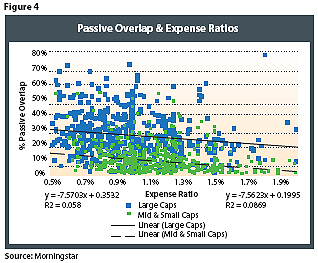# Passive Overlap: Exploring Closet Indexers

October 20, 2009

Ideally, we would expect a negative relationship between passive overlap and expense ratio, where funds with higher levels of passive overlap (i.e., are more indexlike) have lower expense ratios. Figure 4 illustrates the respective expense ratios and Passive Overlap percentages for the test funds for the large-cap category and the mid-cap and small-cap categories (combined).While the regression coefficient was negative for both regressions, the coefficient of determination (R²) was low for both, suggesting there is little relationship (in the aggregate) between expense ratio and passive overlap. Note, though, that the level of passive overlap was considerably higher for the large-cap funds versus the mid-cap and small-cap funds, which is demonstrated by a higher intercept of 0.35 (versus 0.20 for the mid-cap and small-cap funds).

Cost Of Active Management

Although it is relatively easy to compare the passive overlap between managers to determine which is the “most active,” passive overlap ignores one key aspect of active management: an investment’s expense ratio. One way to determine the true “cost” of active management for an investment would be to allocate the expense ratio of the fund over the amount that does not match its benchmark, i.e., its nonpassive overlap (or its Active Portfolio), minus the cost of investing (i.e., a passive strategy). This could be calculated using the following formula:

Active Portfolio Cost = (Expense Ratio - Cost of Passive Strategy) / (1 - Passive Overlap)

In the calculation, it’s important to subtract the cost of a passive strategy from the expense ratio since this value represents the minimum amount it would cost to be invested in the market. Doing so also allows someone to calculate the Active Portfolio Cost of different index or enhanced index funds, which tend to have denominators close to zero due to the high level of passive overlap.

The Active Portfolio Cost formula enables someone to determine the relative cost of different active managers. For example, let’s assume you are choosing between two funds: Fund 1 has an expense ratio of 80 bps and a passive overlap of 50 percent, and Fund 2 has a cost of 100 bps and a passive overlap of 10 percent. In the absence of any information on passive overlap, assuming an investor was indifferent between Fund 1 and Fund 2, he or she would likely select Fund 1 since it has an expense ratio (or management fee) that is 20 bps cheaper. However, when you calculate the Active Portfolio Cost, assuming a 20 bps passive strategy cost, the “cost” of Fund 1 is 120 bps, while the cost of Fund 2 is 89 bps. In other words, Fund 2 is cheaper than Fund 1 when you consider the portion of the total portfolio that is actively managed.

Let’s extend this analysis to some actual mutual funds tested in the analysis. For example, based entirely on expense ratio, the T. Rowe Price Diversified Mid-Cap Growth Fund (PRDMX) would appear to be relatively cheap. Its expense ratio of 1.16 percent puts it in the 40th percentile of all mid-cap growth funds tested (i.e., it’s cheaper than 60 percent of its peers). However, its passive overlap is 53.67 percent (i.e., a little over half of the portfolio is invested exactly as its Russell Midcap Growth benchmark), creating an Active Portfolio Cost of 2.07 percent. This puts the fund in approximately the 85th percentile of all mid-cap growth funds tested (i.e., it’s more expensive than 85 percent of its peers) and makes it significantly less attractive from an “active value” perspective.